Do you like the article?
Share it with others —
Use new possibilities of MetaTrader 5

#### Similar articles# MQL5 Cookbook: Getting properties of an open hedge position

MetaTrader 5Examples | 8 October 2018, 17:14216 1663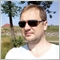### Introduction

In this article, we will discuss the properties of the aggregate position, which is the object of the hedging system.

### 1. Hedge position. Types

A hedge (aggregate) position is a market position, which is formed by several market orders. In the narrow sense, a hedge position includes orders in different directions (buy and sell). However, I propose to use the "hedge" in a broad sense too. Here, a hedge position may also comprise orders in the same direction. This approach is connected with the MetaTrader 5 terminal capabilities: we can open orders in one direction, as well as in different ones.

There are several ways to classify an aggregate position. Perhaps the most popular criterion is distinguishing positions by the type of market orders, which form the aggregate position. So, what orders can form such a position? Table 1 below shows various combinations.

No Type Description
3 Hedge sell Sell operations only
4 Hedge netting sell Net selling
5 Hedge locked Locking (the full hedge)

Table 1. Hedge types

Let's briefly explain these types. If the aggregate position has only buy or only sell orders (in terms adopted in MetaTrader 4), this position will be considered either hedge buy or hedge sell. If the position has different orders (both buy and sell), we will determine which orders are prevailing. If there are more buy orders, the position will be determined as 'hedge netting buy'. If there are more sell orders, this will be a 'hedge netting sell' position. In order to be more precise, we need to deal with the order volumes rather than their number. Suppose, a position has 1 buy order of 1.25 lots and two sell orders of 0.5 and 0.6 lots. The aggregate position will be 'hedge netting buy' with the volume of 0.15 lots:

1.25 – (0.5 + 0.6) = 0.15.

A special kind of mixed position is locking, in which buying and selling balance each other in terms of the trading volume.

Let's formalize these described hedge types in the following enumeration:

```//+------------------------------------------------------------------+
//| Hedge type                                                       |
//+------------------------------------------------------------------+
enum ENUM_HEDGE_TYPE
{
HEDGE_SELL=1,         // sell
HEDGE_NETTING_SELL=3, // netting sell
HEDGE_LOCKED=4,       // lock
};
```

A position in the netting system, which is also aggregate, can only be of one of the two types: either buy or sell. The type identifier is one of the values of the ENUM_POSITION_TYPE enumeration:

2) POSITION_TYPE_SELL.

In the hedging system, we have five types of aggregate positions.

In the next section, we will create a class to handle the hedge position properties.

### 2. The CHedgePositionInfo class

The Standard Library provides the CPositionInfo class, which enables access to the properties of an open market position. We will partially use this class for our case, as the position handled by the class will be presented as a separate market order (in terms of MetaTrader 4). We need a class, which processes all positions within the aggregate position (hedge).

Let's use OOP tools and create the CHedgePositionInfo class:

```//+------------------------------------------------------------------+
//| Class CHedgePositionInfo                                         |
//|              Derives from class CObject.                         |
//+------------------------------------------------------------------+
class CHedgePositionInfo : public CObject
{
//--- === Data members === ---
private:
ENUM_HEDGE_TYPE   m_type;
double            m_volume;
double            m_price;
double            m_stop_loss;
double            m_take_profit;
ulong             m_magic;
//--- objects
CArrayLong        m_tickets;
CSymbolInfo       m_symbol;
CPositionInfo     m_pos_info;

//--- === Methods === ---
public:
//--- constructor/destructor
void              CHedgePositionInfo(void){};
void             ~CHedgePositionInfo(void){};
//--- initialization
bool              Init(const string _symbol,const ulong _magic=0);
//--- get methods
CSymbolInfo      *Symbol(void)       {return GetPointer(m_symbol);};
CArrayLong       *HedgeTickets(void) {return GetPointer(m_tickets);};
CPositionInfo    *PositionInfo(void) {return GetPointer(m_pos_info);};
ulong             Magic(void) const  {return m_magic;};
//--- fast access methods to the integer hedge properties
datetime          Time(void);
ulong             TimeMsc(void);
datetime          TimeUpdate(void);
ulong             TimeUpdateMsc(void);
ENUM_HEDGE_TYPE   HedgeType(void);
//--- fast access methods to the double hedge properties
double            Commission(const bool _full=false);
double            Swap(void);
double            Profit(void);
double            Margin(void);
//--- fast access methods to the string hedge properties
string            TypeDescription(void);
//--- info methods
string            FormatType(string &_str,const uint _type) const;
//--- select
bool              Select(void);
//--- state
void              StoreState(void);
bool              CheckState(void);

private:
//--- calculation methods
bool              AveragePrice(
const SPositionParams &_pos_params,
double &_avg_pr,
double &_base_volume,
double &_quote_volume
);
};
//+------------------------------------------------------------------+
```

A few words about class data members.

First, there is a unique symbol. I.e. a hedge position can include any positions of the same symbol. The symbol is determined based on the m_symbol field, which represents the CSymbolInfo class sample.

Secondly, the magic number (m_magic) can be used to filter orders. The filter enables creation of a position managed by one trading Expert Advisor. This allows creating multiple hedge positions of the same symbol.

There is also a dynamic array for the accounting of orders (m_tickets). Tickets of the hedge position orders will be added to this array.

The functions of obtaining the properties of any selected position (i.e. a market order in terms of MetaTrader 4) are performed by the CPositionInfo class instance (m_pos_info).

Other hedge properties are used for evaluating its state:

• type (m_type);
• volume (m_volume);
• open price (m_price);
• Stop Loss price (m_stop_loss);
• Take Profit price (m_take_profit).

The class construction here is based on the CPositionInfo class logic. This is quite natural. That is why the new class has methods returning integer properties, double properties, etc. And there will be specific methods, of course.

2.1 Initialization method

Before using the class features, we need to initialize the corresponding instance. The method checks whether the EA operates under the "hedging" system conditions and whether the required symbol is selected and/or the magic number is set.

```//+------------------------------------------------------------------+
//| Initialization                                                   |
//+------------------------------------------------------------------+
bool CHedgePositionInfo::Init(const string _symbol,const ulong _magic=0)
{
//--- account margin mode
ENUM_ACCOUNT_MARGIN_MODE margin_mode=(ENUM_ACCOUNT_MARGIN_MODE)AccountInfoInteger(ACCOUNT_MARGIN_MODE);
if(margin_mode!=ACCOUNT_MARGIN_MODE_RETAIL_HEDGING)
{
Print(__FUNCTION__+": no retail hedging!");
return false;
}
if(!m_symbol.Name(_symbol))
{
Print(__FUNCTION__+": a symbol not selected!");
return false;
}
if(symbol_calc_mode!=SYMBOL_CALC_MODE_FOREX)
{
Print(__FUNCTION__+": only for Forex mode!");
return false;
}
m_magic=_magic;
//---
return true;
}
//+------------------------------------------------------------------+
```

This method is mandatory for the subsequent use of the hedge class features. Note that the margin calculation mode is checked in the method. If it does not correspond to "hedging", the method will return false. The contract value calculation method is also checked. We will only work with Forex contracts.

2.2 Integer properties

Integer properties are accessed using the following methods:

1. datetime                   Time(void);
2. ulong                        TimeMsc(void);
3. datetime                   TimeUpdate(void);
4. ulong                        TimeUpdateMsc(void);
5. ENUM_HEDGE_TYPE   HedgeType(void).

Here is the code of the CHedgePositionInfo::Time() method:

```//+------------------------------------------------------------------+
//| Get  the hedge open time                                         |
//+------------------------------------------------------------------+
datetime CHedgePositionInfo::Time(void)
{
datetime hedge_time=WRONG_VALUE;
int hedge_pos_num=m_tickets.Total();
//--- if any positions
if(hedge_pos_num>0)
{
//--- find the first opened position
for(int pos_idx=0;pos_idx<hedge_pos_num;pos_idx++)
{
ulong curr_pos_ticket=m_tickets.At(pos_idx);
if(curr_pos_ticket<LONG_MAX)
if(m_pos_info.SelectByTicket(curr_pos_ticket))
{
datetime curr_pos_time=m_pos_info.Time();
if(curr_pos_time>0)
{
if(hedge_time==0)
hedge_time=curr_pos_time;
else
{
if(curr_pos_time<hedge_time)
hedge_time=curr_pos_time;
}
}
}
}
}
//---
return hedge_time;
}
//+------------------------------------------------------------------+
```

To get the hedge opening time, which is actually the time of the first position within the hedge, we need to go through all its positions and find the earliest one.

In order to get the hedge change time, i.e. of the last changed position, we need to slightly modify the previous method:

```//+------------------------------------------------------------------+
//| Get  the hedge update time                                       |
//+------------------------------------------------------------------+
datetime CHedgePositionInfo::TimeUpdate(void)
{
datetime hedge_time_update=0;
int hedge_pos_num=m_tickets.Total();
//--- if any positions
if(hedge_pos_num>0)
{
//--- find the first opened position
for(int pos_idx=0;pos_idx<hedge_pos_num;pos_idx++)
{
ulong curr_pos_ticket=m_tickets.At(pos_idx);
if(curr_pos_ticket<LONG_MAX)
if(m_pos_info.SelectByTicket(curr_pos_ticket))
{
//--- get the current position update time
datetime curr_pos_time_update=m_pos_info.TimeUpdate();
if(curr_pos_time_update>0)
if(curr_pos_time_update>hedge_time_update)
hedge_time_update=curr_pos_time_update;
}
}
}
//---
return hedge_time_update;
}
//+------------------------------------------------------------------+
```

Here is the code of the method for determining the hedging type:

```//+------------------------------------------------------------------+
//| Get  the hedge type                                              |
//+------------------------------------------------------------------+
ENUM_HEDGE_TYPE CHedgePositionInfo::HedgeType(void)
{
ENUM_HEDGE_TYPE curr_hedge_type=WRONG_VALUE;
int hedge_pos_num=m_tickets.Total();
//--- if any positions
if(hedge_pos_num>0)
{
//--- get the volumes
//--- define a hedge type
{
curr_hedge_type=HEDGE_NETTING_SELL;
else
curr_hedge_type=HEDGE_LOCKED;
}
curr_hedge_type=HEDGE_SELL;
}
//---
return curr_hedge_type;
};
//+------------------------------------------------------------------+
```

The hedge type depends on the difference between the buy and sell volumes. First we check if the position is a hedge in the narrow sense. If the buy and sell volumes are equal, this is the full hedge. If they are not equal, it is a sign of a partial hedge.

Then we check whether the hedge includes volumes of different directions, and not only buy or only sell.

2.3 Double properties

Double properties are accessed using the following methods:

2.    double            PriceOpen(void);
3.    double            StopLoss(void);
4.    double            TakeProfit(void);
5.    double            PriceCurrent(void);
6.    double            Commission(void);
7.    double            Swap(void);
8.    double            Profit(void);
9.    double            Margin(void).

The hedge volume determining method has parameters as references. This implementation enables us to immediately get both the hedge volume and the volume of its components (buy and sell orders).

```//+------------------------------------------------------------------+
//| Get  the hedge volume                                            |
//+------------------------------------------------------------------+
{
double total_vol=0.;
int hedge_pos_num=m_tickets.Total();
//--- if any positions
if(hedge_pos_num>0)
{
for(int pos_idx=0;pos_idx<hedge_pos_num;pos_idx++)
{
ulong curr_pos_ticket=m_tickets.At(pos_idx);
if(curr_pos_ticket<LONG_MAX)
if(m_pos_info.SelectByTicket(curr_pos_ticket))
{
ENUM_POSITION_TYPE curr_pos_type=m_pos_info.PositionType();
double curr_pos_vol=m_pos_info.Volume();
if(curr_pos_vol>0.)
{
//--- else for a sell position
else if(curr_pos_type==POSITION_TYPE_SELL)
_sell_volume+=curr_pos_vol;
}
}
}
}
//---
}
//+------------------------------------------------------------------+
```

An auxiliary CHedgePositionInfo::AveragePrice() method was created for working with price properties. Here is the code block calculating the average hedge price depending on the price level type:

```//--- if the hedge volumes calculated
if(hedge_base_volume!=0. && hedge_quote_volume!=0.)
{
_avg_pr=fabs(hedge_quote_volume/hedge_base_volume);
_base_volume=hedge_base_volume;
_quote_volume=hedge_quote_volume;
return true;
}
```

The value approach is used here: the ratio of the final hedge value in the quote currency to the final hedge value in the deposit currency.

Later, we will analyze such calculation based on a separate example.

Swap and profit obtaining methods sum up the appropriate variables of each position within the hedge, and return the resulting value. The commission obtaining method analyzes deals, which participated in position opening. Here, the parameter allows selecting how to calculate the commission size. Use the default value to calculate commission only based on entry deals. Set the parameter to true if you need to calculate both entry and exit commissions. However, note that the latter option is approximate in nature for several reasons. First, selected positions can be closed by opposite ones. Thus, there will be deals of type DEAL_ENTRY_OUT_BY. Commission is not charged on such deals. Second, if the account currency does not match the base currency, the entry and exit cost may be different if rates change.

```//+------------------------------------------------------------------+
//| Get  the hedge commission                                        |
//+------------------------------------------------------------------+
double CHedgePositionInfo::Commission(const bool _full=false)
{
double hedge_commission=0.;
int hedge_pos_num=m_tickets.Total();
//--- if any positions
if(hedge_pos_num>0)
for(int pos_idx=0;pos_idx<hedge_pos_num;pos_idx++)
{
ulong curr_pos_ticket=m_tickets.At(pos_idx);
if(curr_pos_ticket<LONG_MAX)
if(m_pos_info.SelectByTicket(curr_pos_ticket))
{
long curr_pos_id=m_pos_info.Identifier();
if(curr_pos_id>0)
//--- retrieve the history of deals associated with the selected position
if(HistorySelectByPosition(curr_pos_id))
{
CDealInfo curr_deal;
int deals_num=HistoryDealsTotal();
for(int deal_idx=0;deal_idx<deals_num;deal_idx++)
if(curr_deal.SelectByIndex(deal_idx))
{
ENUM_DEAL_ENTRY curr_deal_entry=curr_deal.Entry();
if(curr_deal_entry==DEAL_ENTRY_IN)
{
double curr_deal_commission=NormalizeDouble(curr_deal.Commission(),2);
if(curr_deal_commission!=0.)
{
double fac=1.;
if(_full) fac=2.;
hedge_commission+=(fac*curr_deal_commission);
}
}
}

}
}
}
//---
return hedge_commission;
}
//+------------------------------------------------------------------+
```

The class contains the CHedgePositionInfo::Margin() method, which allows determining the margin amount for a hedge position. This method was actually the most difficult to program. A separate article can be written, describing how to correctly determine margin for open positions and pending orders.

2.3.1 Hedge position margin

As described by the developer, there are two methods for calculating margin for bi-directional positions. The type used is determined by the broker. The first method uses a basic calculation, and the second one is based on a larger leg.

I have never met the second calculation type. However, it is also implemented in the code. But first, let's consider the first method. It will have a more complex algorithm, which includes the calculation of margin:

1. for the uncovered volume
2. for the hedged (covered) volume (if hedged margin size is specified)
3. for pending orders

In this article, margin is calculated for the Retail Forex, Futures model. Pending order margin calculation is not considered.

Information on the following parameters is required for a complete calculation of hedge position margin:

1. Deposit currency. Accounts normally have the following deposit currencies: USD, EUR, GBP, CHF.
2. Margin currency. Usually, this is equal to the base currency. For example, the margin currency for EURUSD is the euro (EUR); and for the AUDNZD cross pair, it is the Australian dollar (AUD).
3. The leverage.

Also note that the margin value in the balance line of the terminal's Trade tab will be specified in the deposit currency. Therefore, the calculation result should be the margin value in the deposit currency.

Since the margin currency can differ from the deposit currency, there are several calculation options:

1. When the deposit currency is present in the hedge position symbol as the base currency. Let's say you trade USDCHF on a dollar account.
2. When the deposit currency is present in the hedge position symbol as the quoted currency. For example, if you trade EURUSD on a dollar account.
3. When the hedge position symbol does not contain the deposit currency. For example, if you trade AUDNZD on a dollar account.

The first option will be the easiest to calculate, and the last variant is the most difficult one. Now, let us consider calculation examples for each option.

Variant 1

Suppose, there is a dollar account (deposit) with five open USDCHF positions (Fig.1).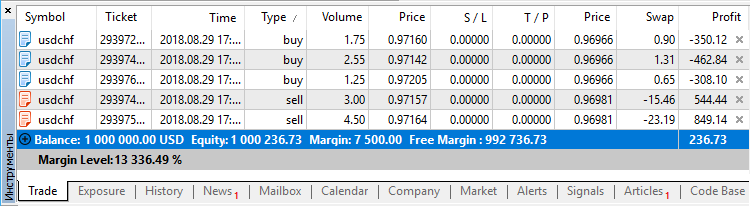Fig.1 Market USDCHF positions

Basic parameters:

Account currency - USD.

Margin currency - USD.

Leverage - 1:100.

Three of them are buy positions. The total volume of positions is 5.55 lots, which is equal to \$555,000. The other two are sell positions. The total volume of these positions is 7.5 lots, which is equal to \$750,000.

A) Calculation for the uncovered volume

The uncovered volume is equal to 1.95 lots, i.e. \$195,000. It is a sell volume, because more was sold than bought. However, buying or selling doesn't matter in our case, because we do not need to calculate the weighted average price.

The margin on this amount is calculated taking into account the leverage:

\$195,000 / 100 = \$1,950.

B) Calculation for the hedged volume

The hedged volume is equal to 5.55 lots, i.e. \$555,000.

The margin on this amount is calculated taking into account the leverage:

\$555,000 / 100 = \$5,550.

The resulting margin value is calculated as the sum of margin for the hedged and uncovered volume.

\$1,950 + \$5,550 = \$7,500.

This is the value displayed in the trading terminal as "Margin".

Variant 2

Now, there is a dollar account (deposit) with five open EURUSD positions (Fig.2).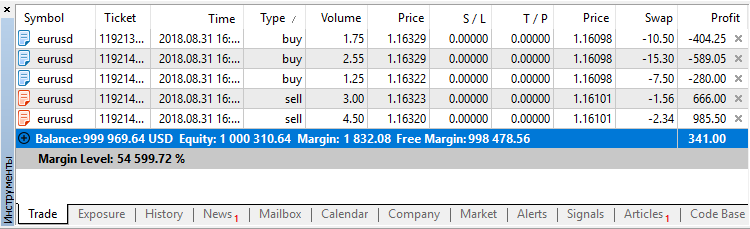Fig.2 Market EURUSD positions

Basic parameters:

• Account currency - USD.
• Margin currency - EUR.
• Leverage - 1:300.

Three of them are buy positions. The total volume of positions is 5.55 lots, which is equal to €555,000 or \$645,617.20. The weighted average price of Buy positions is \$1.163274.

There are also two sell positions. The total volume of these positions is 7.5 lots, which is equal to €750,000 or \$872 409. The average weighted selling price is \$1.163212.

All the positions are presented in Table 2.

Type Volume Price Value, \$
sell 3.00 1.16323 348,969.00
sell 4.50 1.16320 523,440.00
Total 13.05 1.1632385 1,518,026.20

Table 2. Market EURUSD positions

There are 5 positions in total. The total volume of positions is 13.05 lots, which is equal to €1,305,000 or \$1,518,026.20. The average weighted position price is \$1.16324.

A) Calculation for the uncovered volume

The uncovered volume is equal to 1.95 lots, i.e. €195,000. It is a sell volume, because more was sold than bought. So, we use the average weighted selling price to determine the volume cost:

\$1.163212 * €195,000 = \$226,826.34.

The margin on this amount is calculated taking into account the leverage:

\$226,826.34 / 300 = \$756.09.

B) Calculation for the hedged volume

The hedged volume is equal to 5.55 lots, i.e. €555,000. Let's use the average weighted price of all positions to determine the value for this volume:

\$1.1632385 * €555,000 = \$645,597.35.

The margin on this amount is calculated taking into account the leverage:

\$645,597.35 / 300 = \$2,151.99.

Then, in theory, the entire gross margin should be equal to the following:

\$756.09 + \$2,151.99 = \$2,908.08.

However, the value displayed in the terminal is \$1,832.08.

This is because the "Hedged margin" parameter from the symbol specification is taken into account for the covered (hedged) volume. If it is less than the contract size, we get some multiplier. This parameter in our symbols specification is equal to 50000. Then:

Hedged volume value = \$1.1632385 * €555,000 / (100,000 / 50,000) = \$322,798,67.

Margin for the hedged volume = \$322,798.67 / 300 = \$1,076.00.

Calculating the sum: \$756.09 + \$1,076.00 = \$1,832.08. This is the value displayed in the terminal.

Variant 3

This time we deal with a dollar account (deposit), having five open AUDNZD positions (Fig.3).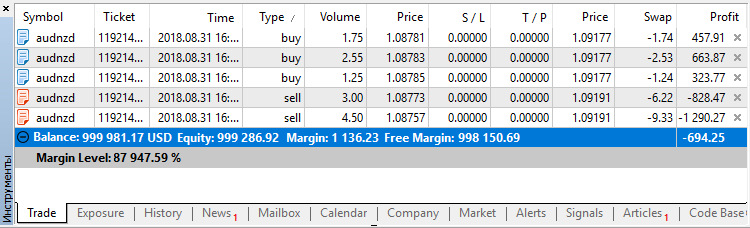Fig.3 Market positions for the AUDNZD cross pair

Basic parameters:

• Account currency - USD.
• Margin currency - AUD.
• Leverage - 1:300.

Three of them are buy positions. The total volume of positions is 5.55 lots, which is equal to A\$555,000 or \$400,442.35. The weighted average price of Buy positions is \$0.7215178.

There are also two sell positions. The total volume of positions is 5.55 lots, which is equal to A\$750,000 or \$541,035.00. The average weighted selling price is \$0.72138.

All the positions are presented in Table 3.

Type Volume Price Value, \$
sell 3.00 0.72144 216,432.00
sell 4.50 0.72134 324,603.00
Total 13.05 0.72144 941,477.35

Table 3. Market positions for the AUDNZD cross pair

The Price column features position open prices not for AUDNZD, but for the AUDUSD symbol. This allows the evaluation of the traded volume in the deposit currency. Here, we need to refer to the tick and quote history of the pair, which includes the deposit currency and the margin currency. Therefore, the calculated values ​​may slightly differ from the actual ones.

There are 5 positions in total. The total volume of positions is 13.05 lots, which is equal to A\$1,305,000 or \$941,477.35. The average weighted position price is \$0.72144.

A) Calculation for the uncovered volume

The uncovered volume is equal to 1.95 lots, i.e. A\$195,000. It is a sell volume, because more was sold than bought. So, we use the average weighted selling price to determine the volume cost:

\$0.72138 * A\$195,000 = \$140,669.10.

The margin on this amount is calculated taking into account the leverage:

\$140,669.10 / 300 = \$468.90.

B) Calculation for the hedged volume

The hedged volume is equal to 5.55 lots, i.e. A\$555,000. Let's use the average weighted price of all positions taking into account the "Hedged margin" parameter to determine the value for this volume:

\$0.72144 * A\$555,000 / (100,000 / 50,000) = \$200,199.21.

The margin on this amount is calculated taking into account the leverage:

\$200,199.21 / 300 = \$667.33.

Calculating the sum: \$468.90 + \$667.33 = \$1,136.23. Compare the result with the Fig.3: it is equal to the value displayed in the terminal.

2.4 Other properties

The class contains methods for working with the hedge state: StoreState() and CheckState(). Similar to a common position, the state includes the type, volume, Open price, Stop Loss and Take Profit prices.

The only text property method TypeDescription() returns the hedge type as a string.

Of particular note is the Select() method, which allows selecting a hedge position. Here is the method code:

```//+------------------------------------------------------------------+
//| Selects hedge positions                                          |
//+------------------------------------------------------------------+
bool CHedgePositionInfo::Select(void)
{
string hedge_symbol=m_symbol.Name();
//--- clear all positions
m_tickets.Shutdown();
//--- collect positions
int pos_num=PositionsTotal();
for(int pos_idx=0;pos_idx<pos_num;pos_idx++)
if(m_pos_info.SelectByIndex(pos_idx))
{
string curr_pos_symbol=m_pos_info.Symbol();
//--- select by symbol
if(!StringCompare(hedge_symbol,curr_pos_symbol))
{
//--- if to select by magic
bool is_the_same_magic=true;
if(m_magic>0)
{
long curr_pos_magic=m_pos_info.Magic();
if(m_magic!=curr_pos_magic)
is_the_same_magic=false;
}
if(is_the_same_magic)
{
ulong curr_pos_ticket=m_pos_info.Ticket();
if(curr_pos_ticket>0)
{
PrintFormat(__FUNCTION__+": failed to add #%d ticket!",curr_pos_ticket);
return false;
}
}
}
}
//---
return m_tickets.Total()>0;
}
//+------------------------------------------------------------------+
```

The main purpose of the method is to update the tickets of the positions that make up the hedge. It deals with the market positions, which have the symbol matching the hedge symbol. The selection can be additionally filtered by the Magic.

### 3. Examples

We have created a class that operates with the hedge position properties. In this section, we will deal with practical examples. Let's start with a simple script.

3.1 A test script

For training purposes, I have created the Test_hedge_properties.mq5 script, which displays hedge properties in the Journal, in the "Experts" tab.

In the first margin calculation variant, we had five USDCHF positions (Fig.1). Run the script. The following information will appear in the Journal:

```2018.09.03 18:51:37.078 Test_hedge_properties (AUDNZD,H1)       ---== Hedge properties==---
2018.09.03 18:51:37.078 Test_hedge_properties (AUDNZD,H1)       Symbol: USDCHF
2018.09.03 18:51:37.078 Test_hedge_properties (AUDNZD,H1)       Positions total = 5
2018.09.03 18:51:37.078 Test_hedge_properties (AUDNZD,H1)               1) #293972991 buy 1.75 USDCHF 0.97160000
2018.09.03 18:51:37.078 Test_hedge_properties (AUDNZD,H1)               2) #293974150 buy 2.55 USDCHF 0.97142000
2018.09.03 18:51:37.078 Test_hedge_properties (AUDNZD,H1)               3) #293974889 sell 3.00 USDCHF 0.97157000
2018.09.03 18:51:37.078 Test_hedge_properties (AUDNZD,H1)               4) #293975329 sell 4.50 USDCHF 0.97164000
2018.09.03 18:51:37.078 Test_hedge_properties (AUDNZD,H1)               5) #293976289 buy 1.25 USDCHF 0.97205000
2018.09.03 18:51:37.078 Test_hedge_properties (AUDNZD,H1)       Magic: 0
2018.09.03 18:51:37.078 Test_hedge_properties (AUDNZD,H1)       Time: 2018.08.29 17:15:44
2018.09.03 18:51:37.078 Test_hedge_properties (AUDNZD,H1)       Time in msc: 1535562944628
2018.09.03 18:51:37.078 Test_hedge_properties (AUDNZD,H1)       Update time: 2018.08.29 17:20:35
2018.09.03 18:51:37.078 Test_hedge_properties (AUDNZD,H1)       Update time in msc: 1535563235034
2018.09.03 18:51:37.078 Test_hedge_properties (AUDNZD,H1)       Type: HEDGE_NETTING_SELL
2018.09.03 18:51:37.078 Test_hedge_properties (AUDNZD,H1)       Type description: hedge netting sell
2018.09.03 18:51:37.078 Test_hedge_properties (AUDNZD,H1)       Volume: -1.95
2018.09.03 18:51:37.078 Test_hedge_properties (AUDNZD,H1)       Buy volume: 5.55
2018.09.03 18:51:37.078 Test_hedge_properties (AUDNZD,H1)       Sell volume: 7.50
2018.09.03 18:51:37.078 Test_hedge_properties (AUDNZD,H1)       Open price: 0.97159
2018.09.03 18:51:37.078 Test_hedge_properties (AUDNZD,H1)       Sl-price: -1.00000
2018.09.03 18:51:37.078 Test_hedge_properties (AUDNZD,H1)       Tp-price: -1.00000
2018.09.03 18:51:37.078 Test_hedge_properties (AUDNZD,H1)       Current price: 0.96956
2018.09.03 18:51:37.078 Test_hedge_properties (AUDNZD,H1)       Commission: 0.00
2018.09.03 18:51:37.078 Test_hedge_properties (AUDNZD,H1)       Swap: -35.79
2018.09.03 18:51:37.078 Test_hedge_properties (AUDNZD,H1)       Profit: 409.77
2018.09.03 18:51:37.078 Test_hedge_properties (AUDNZD,H1)       Margin: 7500.00

```

In the second variant, we dealt with the properties of the EURUSD position (Fig.2). The following information was obtained after running the script:

```2018.09.03 18:55:09.469 Test_hedge_properties (AUDNZD,H1)       ---== Hedge properties==---
2018.09.03 18:55:09.469 Test_hedge_properties (AUDNZD,H1)       Symbol: EURUSD
2018.09.03 18:55:09.469 Test_hedge_properties (AUDNZD,H1)       Positions total = 5
2018.09.03 18:55:09.469 Test_hedge_properties (AUDNZD,H1)               1) #119213986 buy 1.75 EURUSD 1.16329000
2018.09.03 18:55:09.469 Test_hedge_properties (AUDNZD,H1)               2) #119214003 buy 2.55 EURUSD 1.16329000
2018.09.03 18:55:09.469 Test_hedge_properties (AUDNZD,H1)               3) #119214004 buy 1.25 EURUSD 1.16322000
2018.09.03 18:55:09.469 Test_hedge_properties (AUDNZD,H1)               4) #119214011 sell 3.00 EURUSD 1.16323000
2018.09.03 18:55:09.469 Test_hedge_properties (AUDNZD,H1)               5) #119214021 sell 4.50 EURUSD 1.16320000
2018.09.03 18:55:09.469 Test_hedge_properties (AUDNZD,H1)       Magic: 0
2018.09.03 18:55:09.469 Test_hedge_properties (AUDNZD,H1)       Time: 2018.08.31 16:38:10
2018.09.03 18:55:09.469 Test_hedge_properties (AUDNZD,H1)       Time in msc: 1535733490531
2018.09.03 18:55:09.469 Test_hedge_properties (AUDNZD,H1)       Update time: 2018.08.31 16:38:49
2018.09.03 18:55:09.469 Test_hedge_properties (AUDNZD,H1)       Update time in msc: 1535733529678
2018.09.03 18:55:09.469 Test_hedge_properties (AUDNZD,H1)       Type: HEDGE_NETTING_SELL
2018.09.03 18:55:09.469 Test_hedge_properties (AUDNZD,H1)       Type description: hedge netting sell
2018.09.03 18:55:09.469 Test_hedge_properties (AUDNZD,H1)       Volume: -1.95
2018.09.03 18:55:09.469 Test_hedge_properties (AUDNZD,H1)       Buy volume: 5.55
2018.09.03 18:55:09.469 Test_hedge_properties (AUDNZD,H1)       Sell volume: 7.50
2018.09.03 18:55:09.469 Test_hedge_properties (AUDNZD,H1)       Open price: 1.16303
2018.09.03 18:55:09.469 Test_hedge_properties (AUDNZD,H1)       Sl-price: -1.00000
2018.09.03 18:55:09.469 Test_hedge_properties (AUDNZD,H1)       Tp-price: -1.00000
2018.09.03 18:55:09.469 Test_hedge_properties (AUDNZD,H1)       Current price: 1.16198
2018.09.03 18:55:09.469 Test_hedge_properties (AUDNZD,H1)       Commission: 0.00
2018.09.03 18:55:09.469 Test_hedge_properties (AUDNZD,H1)       Swap: -37.20
2018.09.03 18:55:09.469 Test_hedge_properties (AUDNZD,H1)       Profit: 206.60
2018.09.03 18:55:09.469 Test_hedge_properties (AUDNZD,H1)       Margin: 1832.08

```

In the first variant, we had AUDNZD positions (Fig.3). The script prints the following information to the Journal:

```2018.09.03 18:47:25.369 Test_hedge_properties (EURUSD,H1)       ---== Hedge properties==---
2018.09.03 18:47:25.369 Test_hedge_properties (EURUSD,H1)       Symbol: AUDNZD
2018.09.03 18:47:25.369 Test_hedge_properties (EURUSD,H1)       Positions total = 5
2018.09.03 18:47:25.369 Test_hedge_properties (EURUSD,H1)               1) #119214062 buy 1.75 AUDNZD 1.08781000
2018.09.03 18:47:25.369 Test_hedge_properties (EURUSD,H1)               2) #119214068 buy 2.55 AUDNZD 1.08783000
2018.09.03 18:47:25.369 Test_hedge_properties (EURUSD,H1)               3) #119214071 buy 1.25 AUDNZD 1.08785000
2018.09.03 18:47:25.369 Test_hedge_properties (EURUSD,H1)               4) #119214083 sell 3.00 AUDNZD 1.08773000
2018.09.03 18:47:25.369 Test_hedge_properties (EURUSD,H1)               5) #119214092 sell 4.50 AUDNZD 1.08757000
2018.09.03 18:47:25.369 Test_hedge_properties (EURUSD,H1)       Magic: 0
2018.09.03 18:47:25.369 Test_hedge_properties (EURUSD,H1)       Time: 2018.08.31 16:39:41
2018.09.03 18:47:25.369 Test_hedge_properties (EURUSD,H1)       Time in msc: 1535733581113
2018.09.03 18:47:25.369 Test_hedge_properties (EURUSD,H1)       Update time: 2018.08.31 16:40:07
2018.09.03 18:47:25.369 Test_hedge_properties (EURUSD,H1)       Update time in msc: 1535733607241
2018.09.03 18:47:25.369 Test_hedge_properties (EURUSD,H1)       Type: HEDGE_NETTING_SELL
2018.09.03 18:47:25.369 Test_hedge_properties (EURUSD,H1)       Type description: hedge netting sell
2018.09.03 18:47:25.369 Test_hedge_properties (EURUSD,H1)       Volume: -1.95
2018.09.03 18:47:25.369 Test_hedge_properties (EURUSD,H1)       Buy volume: 5.55
2018.09.03 18:47:25.369 Test_hedge_properties (EURUSD,H1)       Sell volume: 7.50
2018.09.03 18:47:25.369 Test_hedge_properties (EURUSD,H1)       Open price: 1.08708
2018.09.03 18:47:25.369 Test_hedge_properties (EURUSD,H1)       Sl-price: -1.00000
2018.09.03 18:47:25.369 Test_hedge_properties (EURUSD,H1)       Tp-price: -1.00000
2018.09.03 18:47:25.369 Test_hedge_properties (EURUSD,H1)       Current price: 1.09314
2018.09.03 18:47:25.369 Test_hedge_properties (EURUSD,H1)       Commission: 0.00
2018.09.03 18:47:25.369 Test_hedge_properties (EURUSD,H1)       Swap: -21.06
2018.09.03 18:47:25.369 Test_hedge_properties (EURUSD,H1)       Profit: -779.45
2018.09.03 18:47:25.369 Test_hedge_properties (EURUSD,H1)       Margin: 1136.23

```

3.1 Hedge Properties panel

Now we will complicate the task. Using the Standard Library, we will create the HedgePropertiesEA.mq5 Expert Advisor, which draws a panel on the chart, displaying the properties of the selected hedge position.

For these purposes, let's create the CHedgeDialog class derived from the standard CAppDialog class. This class will help us avoid the need to program typical tasks, such as minimizing and maximizing the panel window, handling changes in the panel elements, etc.

```//+------------------------------------------------------------------+
//| Class CHedgeDialog                                               |
//| Purpose: Class for displaying a hedge position info.             |
//|              Derives from class CAppDialog.                      |
//+------------------------------------------------------------------+
class CHedgeDialog : private CAppDialog
{
//--- === Data members === ---
private:
CArrayString      m_symbols_arr;
//--- controls
CLabel            m_labels[FIELDS_NUM+1];
CEdit             m_edits[FIELDS_NUM];
CComboBox         m_combo;
bool              m_to_refresh;
//--- === Methods === ---
public:
//--- constructor/destructor
void              CHedgeDialog(void) {};
void             ~CHedgeDialog(void) {};
//--- initialization
bool              Init(void);
void              Deinit(const int _reason);
//--- processing
void              OnChartEvent(const int _id,
const long &_lparam,
const double &_dparam,
const string &_sparam);
//---
private:
int               HedgeSymbols(void);
void              RefreshPanel(void);
};
//+------------------------------------------------------------------+
```

The class instance in the EA code will call and handle initialization and deinitialization events, chart events, as well as events associated with trade transactions.

The hedge position properties panel is shown in Fig.4.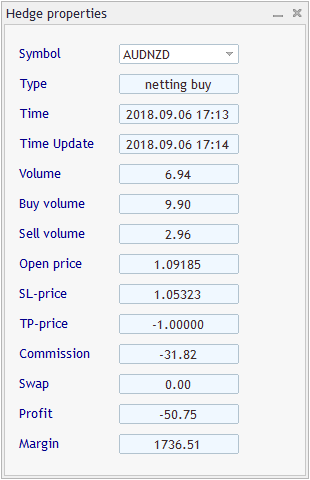Fig.4 Hedge position properties panel

One of the main methods is CHedgeDialog::RefreshPanel(). Whenever needed, it updates the panel information fields. Some difficulty in programing and testing was caused by situations involving a change in the number of hedges. In this case, it is necessary to change the unique symbols in the drop-down list and avoid the infinite loop of the OnChartEvent() handler calls. For this purpose, I used the limit for successive handler calls with a length of 1 sec.

```//--- check the limit for refreshing
if(!m_to_refresh)
{
uint last_cnt=GetTickCount();
static uint prev_cnt=0;
uint msc_elapsed=last_cnt-prev_cnt;
prev_cnt=last_cnt;
if(msc_elapsed>1000)
m_to_refresh=true;
else
return;
}
```

The full code of the HedgePropertiesEA.mq5 Expert Advisor is available in the attached zip.

### Conclusions

In addition to being a multi-asset trading terminal, MetaTrader 5 supports different position management systems. Such opportunities provide significantly expanded options for the implementation and formalization of trading ideas.

Translated from Russian by MetaQuotes Ltd.
Original article: https://www.mql5.com/ru/articles/4830

Attached files |
Hedge.zip (15.37 KB)

#### Other articles by this author

Last comments | Go to discussion (3)| 14 Jul 2019 at 07:09

Hi,

When I downloaded the zip file and extracted it and opened them up in the editor, when I then compiled them they came back with heaps of errors.

Is there anything I can use?

Cheers| 20 Jul 2019 at 21:19

If you follow my way there will be no errors. The way is simple. Open you MetaEditor and:

1) create a subfolder "Hedge" in the folder "Shared Projects";

2) place the source files into the subfolder.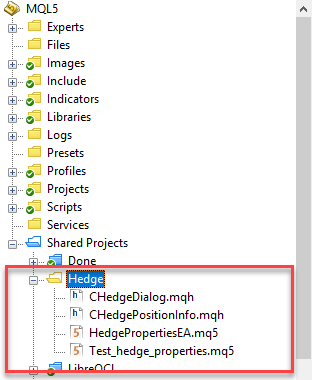Then you may compile HedgePropertiesEA.mq5 (an expert advisor) and  Test_hedge_properties.mq5 (a script). Once the compilation successfully finishes you will find the executables in the terminal navigator.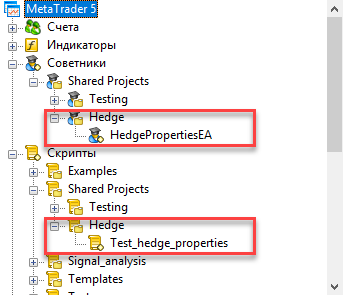| 19 Aug 2019 at 13:23

Hi Denis,

I would like to start by thanking you for this amazing library, it makes life very easy for novice users like myself. I am not a professional programmer, but just about manage to understand, tweak and debug some code a bit.

I am using your sample code from "Test_Hedge_Properties" as a function in an EA that I am testing, and found that after closing all (2) positions, when I call the function

"Update_Hedge_Info", after 1st ticket is closed, the variable that counts the number of positions, "hdg_number_of_pos_total" updates from (2) to (1).

However, after the second ticket closes, the variable still shows (1), it does not update to (0).

I am not sure if I have coded it right, but your kind input and expertise will be greatly appreciated.

I am attaching the EA and screenshot.

Thank you and regards.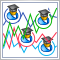Using indicators for optimizing Expert Advisors in real time
Efficiency of any trading robot depends on the correct selection of its parameters (optimization). However, parameters that are considered optimal for one time interval may not retain their effectiveness in another period of trading history. Besides, EAs showing profit during tests turn out to be loss-making in real time. The issue of continuous optimization comes to the fore here. When facing plenty of routine work, humans always look for ways to automate it. In this article, I propose a non-standard approach to solving this issue.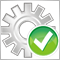Automated Optimization of an EA for MetaTrader 5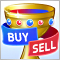Reversing: The holy grail or a dangerous delusion?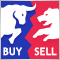Elder-Ray (Bulls Power and Bears Power)